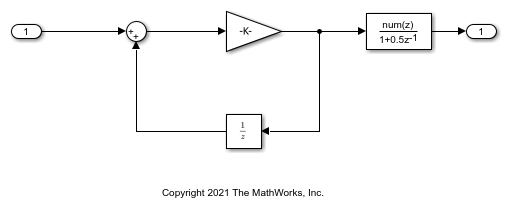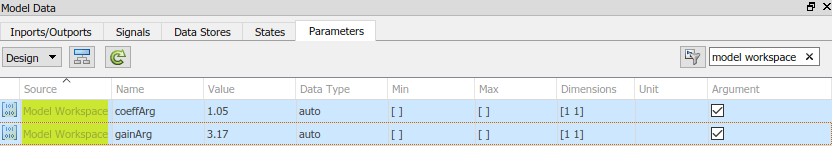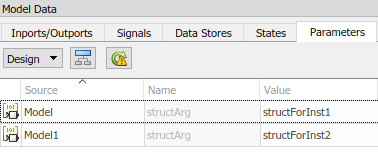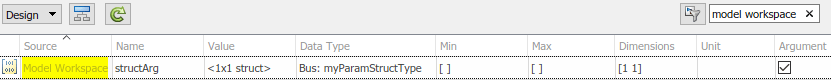## Specify Instance-Specific Parameter Values for Reusable Referenced Model

When you use model referencing to break a large system into components, each component is a separate model. You can reuse a component by referring to it with multiple Model blocks. Each Model block is an instance of the component. You can then configure a block parameter (such as the Gain parameter of a Gain block) to use either the same value or a different value for each instance of the component. To use different values, create and use a model argument to set the value of the block parameter.

When you generate code from a model hierarchy that uses model arguments, the arguments appear in the code as formal parameters of the referenced model entry-point functions, such as the output (`step`) function. The generated code then passes the instance-specific parameter values, which you specify in each Model block, to the corresponding function calls.

Whether you use or do not use model arguments, you can use storage classes to configure block parameters to appear in the generated code as tunable global variables. You can also use storage classes to generate tunable model argument values, which the generated code stores in memory and passes to the function calls. You can then change the values during execution.

### Pass Parameter Data to Referenced Model Entry-Point Functions as Arguments

Configure a referenced model to accept parameter data through formal parameters of the generated model entry-point function. This technique enables you to specify a different parameter value for each instance (Model block) of the referenced model.

Configure Referenced Model to Use Model Arguments

Open the model `ex_arg_code_ref`. This model represents a reusable algorithm.

```open_system('ex_arg_code_ref') ```On the Modeling tab, click Model Data Editor.

In the Model Data editor, in the data table, use the Data Type column to set the data type of the Inport block to `single`. Due to data type inheritance, the other signals in the model use the same data type.

Select the Parameters tab.

In the model, select the Gain block.

In the Model Data Editor, use the Value column to set the value of the Gain parameter to `gainArg`.

Next to `gainArg`, click the action button (3 vertical dots) and select Create.

In the Create New Data dialog box, set Value to `Simulink.Parameter` and Location to `Model Workspace`. Click Create.

In the property dialog box, set Value to a number, for example, `3.17`. Click OK.

Use the Model Data Editor to set the Numerator parameter by using a `Simulink.Parameter` object named `coeffArg` whose value is `1.05`. As with `gainArg`, store the parameter object in the model workspace.

In the Model Data Editor, click the Show/refresh additional information button.

Use the Filter contents box to find each parameter object (`gainArg` and `coeffArg`). For each object, select the check box in the Argument column.Save the `ex_arg_code_ref` model.

Alternatively, at the command prompt, you can use these commands to configure the blocks and the parameter objects:

```set_param('ex_arg_code_ref/In1','OutDataTypeStr','single') set_param('ex_arg_code_ref/Gain','Gain','gainArg') modelWorkspace = get_param('ex_arg_code_ref','ModelWorkspace'); assignin(modelWorkspace,'gainArg',Simulink.Parameter(3.17)); set_param('ex_arg_code_ref/Discrete Filter','Numerator','coeffArg') assignin(modelWorkspace,'coeffArg',Simulink.Parameter(1.05)); set_param('ex_arg_code_ref','ParameterArgumentNames','coeffArg,gainArg') save_system('ex_arg_code_ref') ```

Specify Instance-Specific Parameter Values in Model Blocks

Open the model `ex_arg_code`. This model uses multiple instances (Model blocks) of the reusable algorithm.

```open_system('ex_arg_code') ```In the model, open the Model Data Editor Parameters tab (Modeling > Model Data Editor). The Model Data Editor shows four rows that correspond to the model arguments, `coeffArg` and `gainArg`, that you can specify for the two Model blocks.

Use the Model Data Editor to set values for the model arguments in `Model`. For example, use the values in the figure. For `Model1`, do not specify a value for the model arguments. By default, a model argument uses the last value specified below it in the model hierarchy (indicated by the value `from below`).Alternatively, at the command prompt, you can use this command to set the values for `Model`:

```set_param('ex_arg_code/Model','ParameterArgumentValues',... struct('coeffArg','0.98','gainArg','2.98')) ```

Generate code from the top model.

```rtwbuild('ex_arg_code') ```
```### Starting serial model reference code generation build ### Successfully updated the model reference code generation target for: ex_arg_code_ref ### Starting build procedure for: ex_arg_code ### Successful completion of build procedure for: ex_arg_code Build Summary Code generation targets built: Model Action Rebuild Reason ================================================================================= ex_arg_code_ref Code generated and compiled ex_arg_code_ref.c does not exist. Top model targets built: Model Action Rebuild Reason ============================================================================================ ex_arg_code Code generated and compiled Code generation information file does not exist. 2 of 2 models built (0 models already up to date) Build duration: 0h 0m 32.065s ```

The file `ex_arg_code_ref.c` defines the referenced model entry-point function, `ex_arg_code_ref`. The function has two formal parameters, `rtp_coeffArg` and `rtp_gainArg`, that correspond to the model arguments, `coeffArg` and `gainArg`. The formal parameters use the data type `real32_T`, which corresponds to the data type `single` in Simulink.

```file = fullfile('slprj','grt','ex_arg_code_ref','ex_arg_code_ref.c'); rtwdemodbtype(file,'/* Output and update for referenced model:',... 'real32_T rtp_gainArg)',1,1) ```
```/* Output and update for referenced model: 'ex_arg_code_ref' */ void ex_arg_code_ref(const real32_T *rtu_In1, real32_T *rty_Out1, DW_ex_arg_code_ref_f_T *localDW, real32_T rtp_coeffArg, real32_T rtp_gainArg) ```

The file `ex_arg_code.c` contains the definition of the top model entry-point function, `ex_arg_code`. This function calls the referenced model entry-point function, `ex_arg_code_ref`, and uses the model argument values that you specified (such as `1.11` and `3.34`) as the values of `rtp_coeffArg` and `rtp_gainArg`.

```file = fullfile('ex_arg_code_grt_rtw','ex_arg_code.c'); rtwdemodbtype(file,'/* ModelReference: ''<Root>/Model'' incorporates:',... '1.05F, 3.17F)',1,1) ```
``` /* ModelReference: '<Root>/Model' incorporates: * Inport: '<Root>/In1' * Outport: '<Root>/Out1' */ ex_arg_code_ref(&ex_arg_code_U.In1, &ex_arg_code_Y.Out1, &(ex_arg_code_DW.Model_InstanceData.rtdw), 0.98F, 2.98F); /* ModelReference: '<Root>/Model1' incorporates: * Inport: '<Root>/In1' * Outport: '<Root>/Out2' */ ex_arg_code_ref(&ex_arg_code_U.In1, &ex_arg_code_Y.Out2, &(ex_arg_code_DW.Model1_InstanceData.rtdw), 1.05F, 3.17F); ```

The formal parameters use the data type `real32_T` (`single`) because:

1. The block parameters in `ex_arg_code_ref` determine their data types through internal rules. For example, in the Gain block dialog box, on the Parameter Attributes tab, Parameter data type is set to `Inherit: Inherit via internal rule` (the default). In this case, the internal rule chooses the same data type as the input and output signals, `single`.

2. The model arguments in the model workspace use context-sensitive data typing because the value of the `DataType` property is set to `auto` (the default). With this setting, the model arguments use the same data type as the block parameters, `single`.

3. The formal parameters in the generated code use the same data type as the model arguments, `single`.

Generate Tunable Argument Values

You can configure the instance-specific values in the Model blocks to appear in the generated code as tunable global variables. This technique enables you to store the parameter values for each instance in memory and to tune the values during code execution.

In the top model `ex_arg_code`, select the Model Data Editor Parameters tab.

Use the Model Data Editor to set the values of the model arguments according to this figure.```set_param('ex_arg_code/Model','ParameterArgumentValues',... struct('coeffArg','coeffForInst1','gainArg','gainForInst1')) set_param('ex_arg_code/Model1','ParameterArgumentValues',... struct('coeffArg','coeffForInst2','gainArg','gainForInst2')) ```

View the contents of the `ex_arg_code_ref` model workspace in Model Explorer.

Copy `gainArg` and `coeffArg` from the `ex_arg_code_ref` model workspace to the base workspace.

Rename `gainArg` as `gainForInst1`. Rename `coeffArg` as `coeffForInst1`.

```gainForInst1 = getVariable(modelWorkspace,'gainArg'); gainForInst1 = copy(gainForInst1); coeffForInst1 = getVariable(modelWorkspace,'coeffArg'); coeffForInst1 = copy(coeffForInst1); ```

Copy `gainForInst1` and `coeffForInst1` as `gainForInst2` and `coeffForInst2`.

```gainForInst2 = copy(gainForInst1); coeffForInst2 = copy(coeffForInst1); ```

Set the instance-specific parameter values by using the `Value` property of the parameter objects in the base workspace.

```gainForInst1.Value = 2.98; coeffForInst1.Value = 0.98; gainForInst2.Value = 3.34; coeffForInst2.Value = 1.11; ```

For each new parameter object, on the Code Generation tab, set `StorageClass` to `ExportedGlobal`. This setting causes the parameter objects to appear in the generated code as tunable global variables.

```gainForInst1.StorageClass = 'ExportedGlobal'; coeffForInst1.StorageClass = 'ExportedGlobal'; gainForInst2.StorageClass = 'ExportedGlobal'; coeffForInst2.StorageClass = 'ExportedGlobal'; ```

Generate code from the top model.

```rtwbuild('ex_arg_code') ```
```### Starting serial model reference code generation build ### Model reference code generation target for ex_arg_code_ref is up to date. ### Starting build procedure for: ex_arg_code ### Successful completion of build procedure for: ex_arg_code Build Summary Top model targets built: Model Action Rebuild Reason =========================================================================== ex_arg_code Code generated and compiled Generated code was out of date. 1 of 2 models built (1 models already up to date) Build duration: 0h 0m 16.112s ```

The file `ex_arg_code.c` defines the global variables that correspond to the parameter objects in the base workspace.

```file = fullfile('ex_arg_code_grt_rtw','ex_arg_code.c'); rtwdemodbtype(file,'/* Exported block parameters */',... '/* Block states (default storage) */',1,0) ```
```/* Exported block parameters */ real32_T coeffForInst1 = 0.98F; /* Variable: coeffForInst1 * Referenced by: '<Root>/Model' */ real32_T coeffForInst2 = 1.11F; /* Variable: coeffForInst2 * Referenced by: '<Root>/Model1' */ real32_T gainForInst1 = 2.98F; /* Variable: gainForInst1 * Referenced by: '<Root>/Model' */ real32_T gainForInst2 = 3.34F; /* Variable: gainForInst2 * Referenced by: '<Root>/Model1' */ ```

In each call to `ex_arg_code_ref`, the top model algorithm uses the global variables to set the values of the formal parameters.

```rtwdemodbtype(file,'/* ModelReference: ''<Root>/Model'' incorporates:',... 'gainForInst2);',1,1) ```
``` /* ModelReference: '<Root>/Model' incorporates: * Inport: '<Root>/In1' * Outport: '<Root>/Out1' */ ex_arg_code_ref(&ex_arg_code_U.In1, &ex_arg_code_Y.Out1, &(ex_arg_code_DW.Model_InstanceData.rtdw), coeffForInst1, gainForInst1); /* ModelReference: '<Root>/Model1' incorporates: * Inport: '<Root>/In1' * Outport: '<Root>/Out2' */ ex_arg_code_ref(&ex_arg_code_U.In1, &ex_arg_code_Y.Out2, &(ex_arg_code_DW.Model1_InstanceData.rtdw), coeffForInst2, gainForInst2); ```

The global variables in the generated code use the data type `real32_T` (`single`) because:

1. The parameter objects in the base workspace use context-sensitive data typing because the `DataType` property is set to `auto` (the default). With this setting, the parameter objects in the base workspace use the same data type as the model arguments, `single`.

2. The global variables in the generated code use the same data type as the parameter objects in the base workspace.

Group Multiple Model Arguments into Single Structure

Use the Model Explorer to copy `gainArg` and `coeffArg` from the `ex_arg_code_ref` model workspace into the base workspace.

```temp = getVariable(modelWorkspace,'gainArg'); gainArg = copy(temp); temp = getVariable(modelWorkspace,'coeffArg'); coeffArg = copy(temp); ```

At the command prompt, combine these two parameter objects into a structure, `structArg`.

```structArg = Simulink.Parameter(struct('gain',gainArg.Value,... 'coeff',coeffArg.Value)); ```

Use the Model Explorer to move `structArg` into the model workspace.

```assignin(modelWorkspace,'structArg',copy(structArg)); clear structArg gainArg coeffArg ```

In the Contents pane, configure `structArg` as the only model argument.

```set_param('ex_arg_code_ref','ParameterArgumentNames','structArg') ```In the `ex_arg_code_ref` model, select the Model Data Editor Parameters tab.

Use the Model Data Editor to set the value of the Gain parameter to `structArg.gain` and the value of the Numerator parameter to `structArg.coeff`. Save the model.

```set_param('ex_arg_code_ref/Gain','Gain','structArg.gain') set_param('ex_arg_code_ref/Discrete Filter',... 'Numerator','structArg.coeff') save_system('ex_arg_code_ref') ```

At the command prompt, combine the four parameter objects in the base workspace into two structures. Each structure stores the parameter values for one instance of `ex_arg_code_ref`.

```structForInst1 = Simulink.Parameter(struct('gain',gainForInst1.Value,... 'coeff',coeffForInst1.Value)); structForInst2 = Simulink.Parameter(struct('gain',gainForInst2.Value,... 'coeff',coeffForInst2.Value)); ```

In the top model ex_arg_code, use the Model Data Editor to set the argument values according to this figure.```set_param('ex_arg_code/Model','ParameterArgumentValues',... struct('structArg','structForInst1')) set_param('ex_arg_code/Model1','ParameterArgumentValues',... struct('structArg','structForInst2')) ```

Click the Show/refresh additional information button.

For the new parameter objects `structForInst1` and `structForInst2`, use the Model Explorer to apply the storage class `ExportedGlobal`.

```structForInst1.StorageClass = 'ExportedGlobal'; structForInst2.StorageClass = 'ExportedGlobal'; ```

At the command prompt, use the function `Simulink.Bus.createObject` to create a `Simulink.Bus` object. The hierarchy of elements in the object matches the hierarchy of the structure fields. The default name of the object is `slBus1`.

```Simulink.Bus.createObject(structForInst1.Value); ```

Rename the bus object as `myParamStructType` by copying it.

```myParamStructType = copy(slBus1); ```

In the Model Data Editor for `ex_arg_code`, use the Data Type column to set the data type of `structForInst1` and `structForInst2` to `Bus: myParamStructType`.

```structForInst1.DataType = 'Bus: myParamStructType'; structForInst2.DataType = 'Bus: myParamStructType'; ``````temp = getVariable(modelWorkspace,'structArg'); temp = copy(temp); temp.DataType = 'Bus: myParamStructType'; assignin(modelWorkspace,'structArg',copy(temp)); ```

Save the `ex_arg_code_ref` model.

```save_system('ex_arg_code_ref') ```

When you use structures to group parameter values, you cannot take advantage of context-sensitive data typing to control the data types of the fields of the structures (for example, the fields of `structForInst1`). However, you can use the properties of the bus object to control the field data types.

At the command promt, set the data type of the elements in the bus object to `single`. The corresponding fields in the structures (such as `structForInst1` and `structArg`) use the same data type.

```myParamStructType.Elements(1).DataType = 'single'; myParamStructType.Elements(2).DataType = 'single'; ```

Generate code from the top model, `ex_arg_code`.

```rtwbuild('ex_arg_code') ```
```### Starting serial model reference code generation build ### Successfully updated the model reference code generation target for: ex_arg_code_ref ### Starting build procedure for: ex_arg_code ### Successful completion of build procedure for: ex_arg_code Build Summary Code generation targets built: Model Action Rebuild Reason ============================================================================================= ex_arg_code_ref Code generated and compiled Model or library ex_arg_code_ref has changed. Top model targets built: Model Action Rebuild Reason =========================================================================== ex_arg_code Code generated and compiled Referenced models were updated. 2 of 2 models built (0 models already up to date) Build duration: 0h 0m 23.374s ```

The file `ex_arg_code_types.h` defines the structure type `myParamStructType`, which corresponds to the `Simulink.Bus` object.

```file = fullfile('ex_arg_code_grt_rtw','ex_arg_code_types.h'); rtwdemodbtype(file,'typedef struct {','} myParamStructType;',1,1) ```
```typedef struct { real32_T gain; real32_T coeff; } myParamStructType; ```

In the file `ex_arg_code_ref.c`, the referenced model entry-point function has a formal parameter, `rtp_structArg`, that correspond to the model argument `structArg`.

```file = fullfile('slprj','grt','ex_arg_code_ref','ex_arg_code_ref.c'); rtwdemodbtype(file,'/* Output and update for referenced model:',... '*rtp_structArg)',1,1) ```
```/* Output and update for referenced model: 'ex_arg_code_ref' */ void ex_arg_code_ref(const real32_T *rtu_In1, real32_T *rty_Out1, DW_ex_arg_code_ref_f_T *localDW, const myParamStructType *rtp_structArg) ```

The file `ex_arg_code.c` defines the global structure variables that correspond to the parameter objects in the base workspace.

```file = fullfile('ex_arg_code_grt_rtw','ex_arg_code.c'); rtwdemodbtype(file,'/* Exported block parameters */',... '/* Block states (default storage) */',1,0) ```
```/* Exported block parameters */ myParamStructType structForInst1 = { 2.98F, 0.98F } ; /* Variable: structForInst1 * Referenced by: '<Root>/Model' */ myParamStructType structForInst2 = { 3.34F, 1.11F } ; /* Variable: structForInst2 * Referenced by: '<Root>/Model1' */ ```

The top model algorithm in the file `ex_arg_code.c` passes the addresses of the structure variables to the referenced model entry-point function.

```file = fullfile('ex_arg_code_grt_rtw','ex_arg_code.c'); rtwdemodbtype(file,'/* ModelReference: ''<Root>/Model'' incorporates:',... '&structForInst2);',1,1) ```
``` /* ModelReference: '<Root>/Model' incorporates: * Inport: '<Root>/In1' * Outport: '<Root>/Out1' */ ex_arg_code_ref(&ex_arg_code_U.In1, &ex_arg_code_Y.Out1, &(ex_arg_code_DW.Model_InstanceData.rtdw), &structForInst1); /* ModelReference: '<Root>/Model1' incorporates: * Inport: '<Root>/In1' * Outport: '<Root>/Out2' */ ex_arg_code_ref(&ex_arg_code_U.In1, &ex_arg_code_Y.Out2, &(ex_arg_code_DW.Model1_InstanceData.rtdw), &structForInst2); ```

### Control Data Types of Model Arguments and Argument Values

When you use model arguments, you can apply a data type to:

• The block parameters that use the arguments (for certain blocks, such as those in the Discrete library).

• The arguments in the referenced model workspace.

• The argument values that you specify in Model blocks.

To generate efficient code by eliminating unnecessary typecasts and C shifts, consider using inherited and context-sensitive data typing to match the data types.

• In the model workspace, use a MATLAB® variable whose data type is `double` or a parameter object whose `DataType` property is set to `auto`. In this case, the variable or object uses the same data type as the block parameter.

• When you set the argument values in Model blocks, take advantage of context-sensitive data typing. To set an argument value, use an untyped value.

• A literal number such as `15.23`. Do not use a typed expression such as `single(15.23)`.

• A MATLAB variable whose data type is `double`.

• A `Simulink.Parameter` object whose `DataType` property is set to `auto`.

In these cases, the number, variable, or object uses the same data type as the model argument in the referenced model workspace. If you also configure the model argument to use context-sensitive data typing, you can control the data types of the block parameter, the argument, and the argument value by specifying the type only for the block parameter.

For basic information about controlling parameter data types, see Parameter Data Types in the Generated Code.

#### Use Model Argument in Different Data Type Contexts

If you use a model argument to set multiple block parameter values, and the data types of the block parameters differ, you cannot use context-sensitive data typing (`double` or `auto`) for the argument in the model workspace. You must explicitly specify a data type for the argument. For example, if the argument in the model workspace is a parameter object (such as `Simulink.Parameter`), set the `DataType` property to a value other than `auto`. For more information about this situation, see Reuse Parameter Data in Different Data Type Contexts.

In this case, you can continue to take advantage of context-sensitive data typing to control the data type of the argument values that you specify in Model blocks. Each argument value uses the data type that you specify for the corresponding argument in the model workspace.